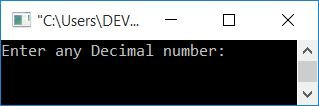# C++ Program to Convert Decimal to Octal

In this article, you will learn and get code on decimal to octal conversion in C++. The program is created with and without using the modulo operator. Also, with and without the use of a user-defined function.

But before going through the program, if you are not aware of the steps and logic used behind the conversion, refer to Decimal to Octal.

## Decimal to Octal in C++

To convert a decimal number to an octal number in C++ programming, you have to ask the user to enter the decimal number first. and then convert it into its equivalent octal value as shown in the program given below:

```#include<iostream>
using namespace std;
int main()
{
int decimalNum, octalNum, i=0;
cout<<"Enter any Decimal number: ";
cin>>decimalNum;
while(decimalNum != 0)
{
octalNum[i] = decimalNum%8;
i++;
decimalNum = decimalNum/8;
}
cout<<"\nEquivalent Octal Value = ";
for(i=(i-1); i>=0; i--)
cout<<octalNum[i];
cout<<endl;
return 0;
}```

This program was built and runs under the Code::Blocks IDE. Here is its sample run:Now, enter any decimal number, such as 358, and press the ENTER key to see its equivalent octal value, as shown in the screenshot below:The following is a dry run of the above program with a decimal number input, say 358:

• Initial value, i=0
• When the user enters a decimal number, say 358, it gets stored in decimalNum.
• Now the condition of the while loop gets evaluated. That is, the condition decimalNum!=0 or 358!=0 evaluates to be true, therefore program flow goes inside the loop.
• There, decimalNum%8 or 358%8 or 6 gets initialized to octalNum[i] or octalNum. Because, we divided the number 358 by 8, we got the remainder of 6.
• The value of i gets incremented. So i=1
• And decimalNum/8, 358/8, or 44 is set to decimalNum.
• Now the program flow goes back and evaluates the condition of the while loop again with a new value of decimalNum.
• Continue evaluating the condition of the while loop until it evaluates to false.
• If we continue the process, we will obtain the following values:
• octalNum=6
• octalNum=4
• octalNum=5
• Before the value of decimalNum becomes equal to 0, the value of i gets incremented. Furthermore, if the value of decimalNum is 0, the while loop condition evaluates to false. As a result, the while loop's evaluation is completed.
• And we must print the value of octalNum[] from the last to the 0th index.
• So, we used the for loop to print the value from i-1 to the 0th index of octalNum[].
• In this way, 546 gets printed as the octal equivalent of the given decimal number 358.

### Decimal to octal conversion in C++ without using the modulo operator

This program does not use the modulo operator (%) while converting a decimal number to octal.

```#include<iostream>
using namespace std;
int main()
{
int decimalNum, octalNum, i=0, temp, chk, rem;
cout<<"Enter any Decimal number: ";
cin>>decimalNum;
while(decimalNum!=0)
{
temp = decimalNum/8;
chk = temp*8;
rem = decimalNum - chk;
octalNum[i] = rem;
i++;
decimalNum = temp;
}
cout<<"\nEquivalent Octal Value = ";
for(i=(i-1); i>=0; i--)
cout<<octalNum[i];
cout<<endl;
return 0;
}```

It will produce the same output as the previous program.

### Decimal to octal conversion in C++ using a user-defined function

This is the last program that uses the user-defined function DecimalToOctal(). It takes a decimal number as its argument and converts it into its equivalent octal value.

```#include<iostream>
using namespace std;
void DecimalToOctal(int);
int octalNum;
static int i;
int main()
{
int decimalNum;
cout<<"Enter any Decimal number: ";
cin>>decimalNum;
DecimalToOctal(decimalNum);
cout<<"\nEquivalent Octal Value = ";
for(i=(i-1); i>=0; i--)
cout<<octalNum[i];
cout<<endl;
return 0;
}
void DecimalToOctal(int decimalNum)
{
while(decimalNum != 0)
{
octalNum[i] = decimalNum%8;
i++;
decimalNum = decimalNum/8;
}
}```

This program has the same output as the first program in this article.

Note: A static int type variable initializes its initial value as 0, automatically.

#### The same program in different languages

C++ Quiz

« Previous Program Next Program »